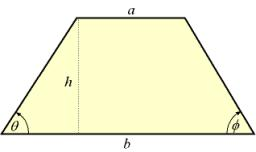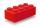# Isosceles trapezoid

Isosceles trapezoid ABCD, AB||CD is given by |CD| = c = 12 cm, height v = 16 cm and |CAB| = 20°. Calculate area of the trapezoid.

S =  703.3542 cm2

### Step-by-step explanation:Did you find an error or inaccuracy? Feel free to write us. Thank you!Tips to related online calculators

#### You need to know the following knowledge to solve this word math problem:

We encourage you to watch this tutorial video on this math problem:

## Related math problems and questions:

• TrapezoidsIn the isosceles trapezoid ABCD we know: AB||CD, |CD| = c = 8 cm, height h = 7 cm, |∠CAB| = 35°. Find the area of the trapezoid.
• Isosceles trapezoidFind the height in an isosceles trapezoid if the area is 520 cm2 and the base a = 25 cm and c = 14 cm. Calculate the interior angles of the trapezoid.
• The isoscelesThe isosceles trapezoid ABCD has bases of 18 cm and 12 cm. The angle at apex A is 60°. What is the circumference and content of the trapezoid?
• A trapezoidA trapezoid with a base length of a = 36.6 cm, with angles α = 60°, β = 48° and the height of the trapezoid is 20 cm. Calculate the lengths of the other sides of the trapezoid.
• CalculateCalculate the area of triangle ABC, if given by alpha = 49°, beta = 31°, and the height on the c side is 9cm.
• The basesThe bases of the isosceles trapezoid ABCD have lengths of 10 cm and 6 cm. Its arms form an angle α = 50˚ with a longer base. Calculate the circumference and content of the ABCD trapezoid.
• BlockCalculate the volume of a cuboid ABCDEFGH if |AB| = 16 cm, |BC| = 19 cm and the angle ∠CDG = 36.9°
• Isosceles trapezoidFind the area of an isosceles trapezoid, if the bases are 12 cm and 20 cm, the length of the arm is 16 cm
• Trapezoid IVIn a trapezoid ABCD (AB||CD) is |AB| = 15cm |CD| = 7 cm, |AC| = 12 cm, AC is perpendicular to BC. What area has a trapezoid ABCD?
• Perimeter of triangleIn the triangle, ABC angle A is 60° angle B is 90°, and side size c is 15 cm. Calculate the triangle circumference.
• Internal anglesThe ABCD is an isosceles trapezoid, which holds: |AB| = 2 |BC| = 2 |CD| = 2 |DA|: On its side BC is a K point such that |BK| = 2 |KC|, on its side CD is the point L such that |CL| = 2 |LD|, and on its side DA the point M is such that | DM | = 2 |MA|. Dete
• IsoscelesIsosceles trapezium ABCD ABC = 12 angle ABC = 40 ° b=6. Calculate the circumference and area.
• Trapezoid MOThe rectangular trapezoid ABCD with the right angle at point B, |AC| = 12, |CD| = 8, diagonals are perpendicular to each other. Calculate the perimeter and area of ​​the trapezoid.
• Ratio in trapeziumThe height v and the base a, c in the trapezoid ABCD are in the ratio 1: 6: 3, its content S = 324 square cm. Peak angle B = 35 degrees. Determine the perimeter of the trapezoid
• FighterA military fighter flies at an altitude of 10 km. The ground position was aimed at an altitude angle of 23° and 12 seconds later at an altitude angle of 27°. Calculate the speed of the fighter in km/h.
• CloudsApproximately at what height is the cloud we see under an angle of 26°10' and see the Sun at an angle of 29°15' and the shade of the cloud is 92 meters away from us?
• Right triangle trigonometricsCalculate the size of the remaining sides and angles of a right triangle ABC if it is given: b = 10 cm; c = 20 cm; angle alpha = 60° and the angle beta = 30° (use the Pythagorean theorem and functions sine, cosine, tangent, cotangent)